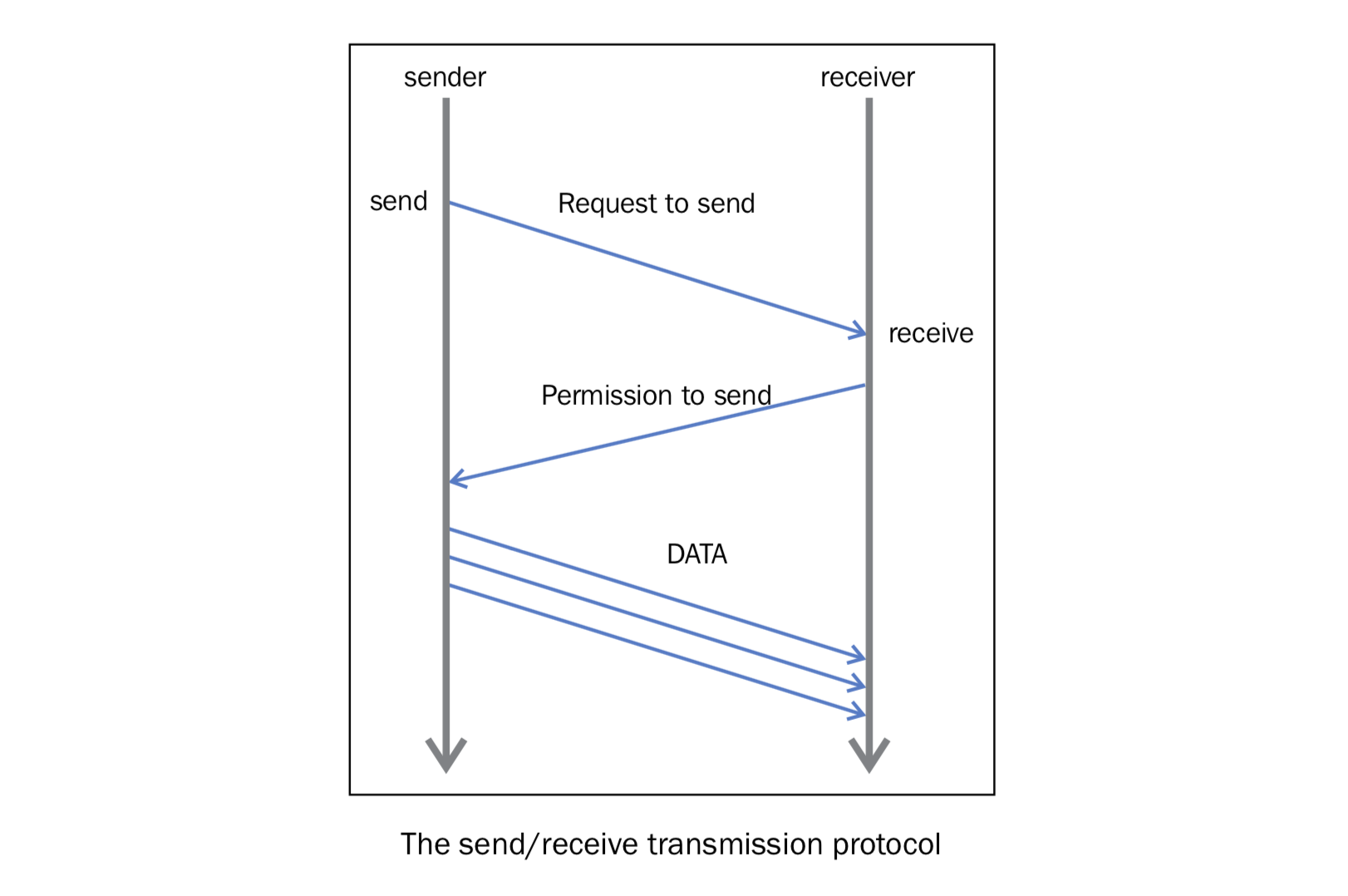# 12. 点对点通讯¶

MPI提供的最实用的一个特性是点对点通讯。两个不同的进程之间可以通过点对点通讯交换数据：一个进程是接收者，一个进程是发送者。

Python的 `mpi4py` 通过下面两个函数提供了点对点通讯功能：

• `Comm.Send(data, process_destination)`: 通过它在交流组中的排名来区分发送给不同进程的数据
• `Comm.Recv(process_source)`: 接收来自源进程的数据，也是通过在交流组中的排名来区分的

`Comm` 变量表示交流者，定义了可以互相通讯的进程组：

```comm = MKPI.COMM_WORLD
```

## 12.1. 如何做…¶

```from mpi4py import MPI
comm = MPI.COMM_WORLD
rank = comm.rank
print("my rank is : " , rank)

if rank == 0:
data = 10000000
destination_process = 4
comm.send(data,dest=destination_process)
print("sending data % s " % data + "to process % d" % destination_process)

if rank == 1:
destination_process = 8
data = "hello"
comm.send(data,dest=destination_process)
print("sending data % s :" % data + "to process % d" % destination_process)

if rank == 4:
data = comm.recv(source = 0)
print("data received is = % s" % data)

if rank == 8:
data1 = comm.recv(source = 1)
print("data1 received is = % s" % data1)
```

```\$ mpiexec -n 9 python pointToPointCommunication.py
```

```('my rank is : ', 5)
('my rank is : ', 1)
sending data hello :to process 8
('my rank is : ', 3)
('my rank is : ', 0)
sending data 10000000 to process 4
('my rank is : ', 2)
('my rank is : ', 7)
('my rank is : ', 4)
('my rank is : ', 8)
('my rank is : ', 6)
```

## 12.2. 讨论¶

```comm = MPI.COMM_WORLD
```

```rank = comm.rand
```

```if rank==0:
data= 10000000
destination_process = 4
comm.send(data,dest=destination_process)
```

```...
if rank == 4:
data = comm.recv(source=0)
```

```if rank==1:
destination_process = 8
data= "hello"
comm.send(data,dest=destination_process)
```

```if rank==8:
data1=comm.recv(source=1)
```## 12.3. 了解更多¶

`comm.send()``comm.recv()` 函数都是阻塞的函数。他们会一直阻塞调用者，知道数据使用完成。同时在MPI中，有两种方式发送和接收数据：

• buffer模式
• 同步模式